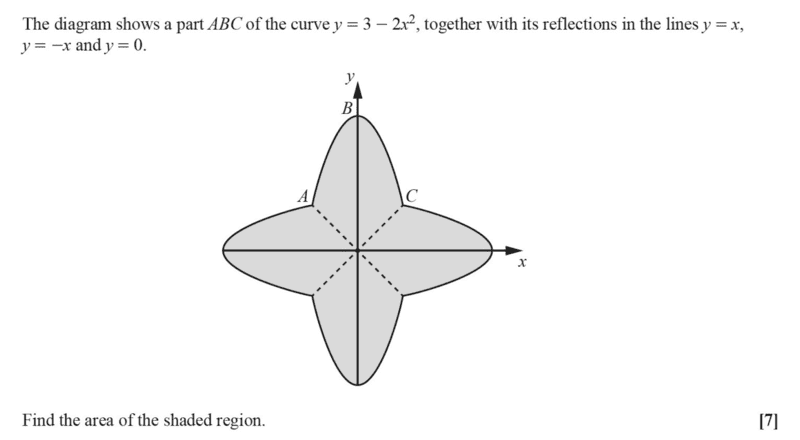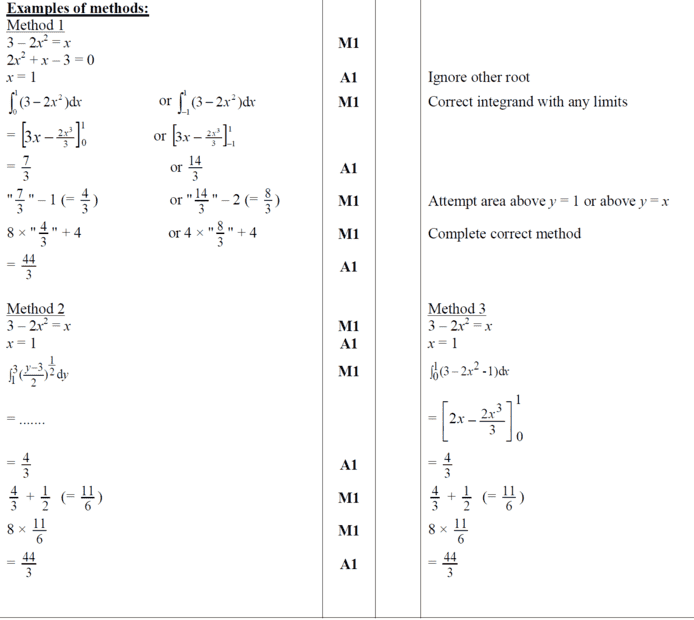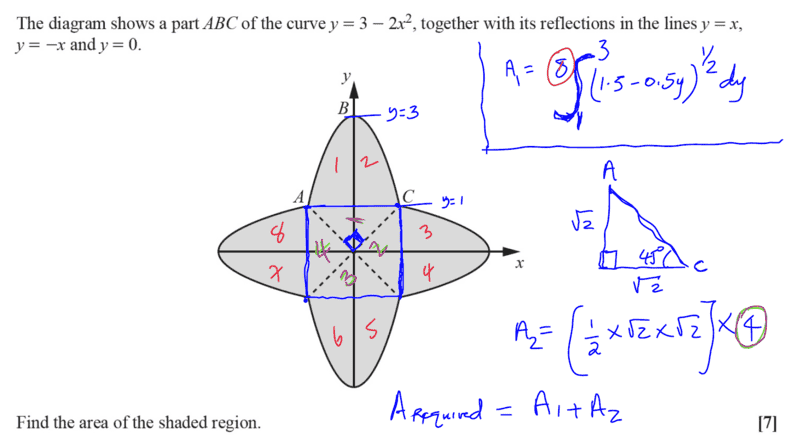# Finding the area under this unusual curve

Gold Member
Homework Statement:
Kindly see attached
Relevant Equations:
A=##\int_a^b f(y)\,dy##
I was looking at the problem below in detail, attached find the problem and the mark scheme solution.Now this was my approach which is just similar to the Mark Scheme method ##2## above where they expressed ##x=f(y)##...

I did it this way;...There was some work involved particularly in expressing ##x=f(y)##...then on the integrating part of

A=8##\int_1^3 (1.5-0.5y)^{0.5}\,dy##
one had to let ##u=1.5-0.5y## to realize ##du=-0.5dy## then proceed from there...##A_{1}=\frac {32} {3}##, and ##A_{2}=4## bingo!

Last edited:

Homework Helper
OK, that seems valid. So now you need to perform the integral.

I'll also note that you could have just observed that ##A_2## is a square of side 2, therefore its area is 4.

•chwala and Leo Liu
Gold Member
OK, that seems valid. So now you need to perform the integral.

I'll also note that you could have just observed that ##A_2## is a square of side 2, therefore its area is 4.
I did not have a question but rather looking at the question in detail... or am i not supposed to share this?i already solved the problem on my own the required area is ##A=A_{1}+A_{2}## as indicated...hence my concluding term, bingo!Homework Helper
Nothing wrong with sharing your solution, but if you post in a forum and expect people to respond, it's generally customary to indicate what sort of response you're looking for.

•chwala
Gold Member
Nothing wrong with sharing your solution, but if you post in a forum and expect people to respond, it's generally customary to indicate what sort of response you're looking for.
Thanks , I've taken note of that...cheers Rpinpa.

Gold Member
OK, that seems valid. So now you need to perform the integral.

I'll also note that you could have just observed that ##A_2## is a square of side 2, therefore its area is 4.
Maybe i should have asked whether the approach i used would realize the full marks...i know that examiners are supposed to stick solely to given mark scheme guides to the latter. Thanks.

Homework Helper
Gold Member
2022 Award
Maybe i should have asked whether the approach i used would realize the full marks...i know that examiners are supposed to stick solely to given mark scheme guides to the latter. Thanks.
I would say you made life hard for yourself by handling the parabolic area in terms of y, dy instead of x, dx. I presume that in your submitted solution you would have shown all your steps, making it quite long.

It is a bit worrying that the question setter deemed it necessary to write out so many variations of the solution for the benefit of the marker. It suggests the markers are not to be trusted to recognise other valid solutions, in which case your radically different solution could indeed cost marks.

•chwala
Mentor
I would say you made life hard for yourself by handling the parabolic area in terms of y, dy instead of x, dx.
I agree.

Due to symmetry, it suffices to calculate the area bounded by points B, C, and O, and then multiply that number by 8. In my work below, I don't show the calculation for the point of intersection C, which is (1, 1).

Area of region bounded by B, C, and O:
##\int_0^1 3 - 2x^2 - x ~dx = \left. 3x - \frac 2 3 x^3 - \frac {x^2} 2 \right|_0^1 = 3 - \frac 2 3 - \frac 1 2 = \frac {11} 6##
Multiply by 8 to get ##\frac {88}6 = \frac{44} 3##

•chwala